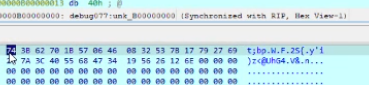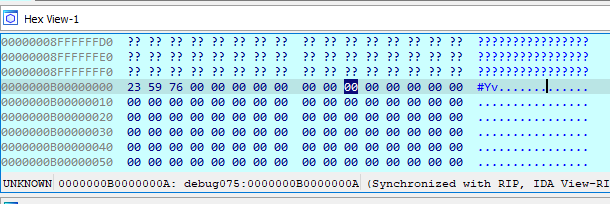# UIUCTF 2021 - smorga's bored

##### by not_really

smorga was bored and wrote a lil cuda chal 4

HINT: CUDA binary utilities

HINT: Use cuobjdump to dump the PTX

\$75 bounty to first solve

Author: Vanilla

I tried running this challenge at the beginning of the CTF, but it turns out that it doesn’t run unless you have an Nvidia GPU.

``````>smorga
hi im smorga..... im bored........ will u play with me?
hello
Cuda error! CUDA driver version is insufficient for CUDA runtime version

>sick
'sick' is not recognized as an internal or external command,
operable program or batch file.
``````

Even when one teammate with an Nvidia GPU tried running it, it still failed.

At this point, the cuobjdump hint was not there, and I moved onto different challenges. In the last hour of the CTF, I found another teammate who was able to run it. I was hoping that it was something like a simple XOR that I could guess, but sadly one character change would affect the rest of the encrypted string, so I downloaded the Nvidia tools and disassembled the program.Debugging over Discord is always fun

## The Program

There’s not much on the C side of things. `FUN_140001058` runs the string encrypting code on the CUDA side and compares it to a string.

``````bool FUN_14000115c(char* param_1) {
char* local_res10;

int iVar1 = cudaMallocManaged(&local_res10, 64, 1);
if (iVar1 == 0) {
strcpy(local_res10, param_1);
//...
if (iVar1 == 0) {
FUN_140001058((ulonglong)local_res10,127);
}
if (iVar1 == 0) {
iVar1 = strcmp(local_res10,"k|\npU\x1e|\n=\r*p.\x13]4v34BIfI}>|\x10 @");
int iVar2 = cudaFree(local_res10);
if (iVar2 == 0) {
return iVar1 == 0;
}
}
}
//...
}

void main(void) {
char local_58;

printf("hi im smorga..... im bored........ will u play with me?\n");
FILE* _File = (FILE *)__acrt_iob_func(0);
fgets(local_58, 64, _File);
int iVar1 = cudaCode(local_58);
char* pcVar2 = "OMG!!! i love u <33\n";
if (iVar1 == 0) {
pcVar2 = "RARRRRR..... i thoght not :\'-(\n";
}
printf(pcVar2);
return;
}
``````

## The Function

After dumping the code with cuobjdump, we get a long function. Nvidia has decent docs here for the assembly.

``````.visible .entry _Z6encodePci(
.param .u64 _Z6encodePci_param_0,
.param .u32 _Z6encodePci_param_1
)
{
.reg .pred %p<21>;
.reg .b16 %rs<2>;
.reg .b32 %r<181>;
.reg .b64 %rd<11>;

.shared .align 1 .b8 _ZZ6encodePciE4temp;

ld.param.u64 %rd4, [_Z6encodePci_param_0];   //rd4 = param0
ld.param.u32 %r61, [_Z6encodePci_param_1];   //r61 = param1
cvta.to.global.u64 %rd10, %rd4;              //rd10 = cvtToGlobal(rd4)
``````

The first bit seems to read from param0 and 1. `cvta.to.global.u64` is used on param0, which according to the docs, “converts address to global space.” This just seems to be general purpose memory. param0 is the input and param1 is 127 (see the `FUN_14000115c` call).

``````mov.u32 %r159, 0;                //r159 = 0

BB0_1:
mov.u32 %r1, %r159;              //r1 = r159
cvt.s64.s32     %rd5, %r1;       //rd5 = r1
add.s64 %rd6, %rd10, %rd5;       //rd6 = rd10+rd5
ld.global.u8 %rs1, [%rd6];       //rs1 = (u8)rd10[rd5]
add.s32 %r159, %r1, 1;           //r159 = r1 + 1
setp.ne.s16     %p1, %rs1, 0;    //p1 = (rs1 == 0)
@%p1 bra BB0_1;
``````

In this block, the code calculates the length of the string and stores it in r1 by comparing each byte in `param0` (rd10) with 0 (null byte).

``````mov.u32 %r3, %tid.x;    //r3 = threadId
add.s32 %r4, %r61, -1;  //r4 = r61 - 1
mov.u32 %r161, 0;       //r161 = 0
mov.u32 %r160, 42;      //r160 = 42
``````

r3 is set to `tid.x` which according to the documentation is the thread ID. It looks like it runs multiple threads. r4 is set to `param1-1`, and r161 and r160 are set to 0 and 42, respectively.

``````BB0_3:
setp.gt.u32     %p2, %r161, %r3; //p2 == (r161 > r3)
@%p2 bra BB0_5;

ld.global.u8 %r65, [%rd10];      //r65 = (u8)rd10
mul.lo.s32 %r66, %r65, %r160;    //r66 = r65 * r160
rem.s32 %r67, %r66, %r61;        //r67 = r66 % r61
add.s32 %r68, %r67, 7;           //r68 = r67 + 7
rem.s32 %r69, %r68, %r4;         //r69 = r68 + r4
add.s32 %r160, %r69, 1;          //r160 = r69 + 1

...

BB0_17:
add.s32 %r27, %r24, 1;           //r27 = r24 + 1
setp.gt.u32     %p9, %r27, %r3;  //p9 = (r27 == r3)
@%p9 bra BB0_19;

ld.global.u8 %r100, [%rd10+7];   //r100 = (u8)rd10
mul.lo.s32 %r101, %r100, %r160;  //r101 = r100 * r160
rem.s32 %r102, %r101, %r61;      //r102 = r101 % r61
add.s32 %r103, %r102, 7;         //r103 = r102 + 7
rem.s32 %r104, %r103, %r4;       //r104 = r103 % r4
add.s32 %r160, %r104, 1;         //r160 = r104 + 1

BB0_19:
add.s64 %rd10, %rd10, 8;         //rd10 = rd10 + 8
add.s32 %r161, %r27, 1;          //r161 = r27 + 1
setp.ne.s32     %p10, %r161, 64; //p10 = (r161 == 64)
@%p10 bra BB0_3;
``````

In this block, we have some add and remainder operations on each character. It does them 8 at a time – probably optimization – but the operation done on each is the same every time. There’s one catch, and it’s that if the thread index is higher than the index of the character it’s checking, it skips it (i.e. thread 0 can only read one character, thread 1 can only read two characters, etc.).

``````meme = 42
idx = 0
while (inpPos < 64)
meme = (((inp[inpPos]*meme)%param1) + 7 + (param1-1) + 1)
break
``````

Next block:

``````mov.u32 %r105, _ZZ6encodePciE4temp; //r105 = temp
add.s32 %r106, %r105, %r3;          //r106 = r105 + r3
st.shared.u8 [%r106], %r160;        //temp[r3] = (u8)r160
setp.ge.u32     %p11, %r3, %r1;     //p11 = (r3 == r1)
@%p11 bra BB0_40; //jumps to exit
``````

Here, it sets `temp[threadIdx] = meme`. Then, it does `bar.sync 0` which, according to the docs, waits for all threads to get to this point before continuing.

The rest is the same add and remainder stuff but backwards but with different constants (69 funny number) so I won’t paste it here.

Last block:

``````cvta.to.global.u64 %rd7, %rd4; //rd7 = cvtToGlobal(param0)
cvt.u64.u32     %rd8, %r3;     //rd8 = r3
add.s64 %rd9, %rd7, %rd8;      //rd9 = rd7 + rd8
st.global.u8 [%rd9], %r171;    //rd7[r3] = r171
``````

It finally ends with setting `param0[threadId] = meme` for each thread.

This code in Python looks like this:

``````inp = [0x61,0x61]

strLen = len(inp)

tmp = *strLen
final = *strLen

param1 = 0x7f

for i in range(strLen):
meme = 42
for j in range(i+1):
meme = (((((inp[j]*meme)%param1) + 7) % (param1-1)) + 1)
tmp[i] = meme&0xff

for i in range(strLen):
meme = 69
for j in range(i+1):
meme = (((((tmp[strLen-1-j]*meme)%param1) + 39) % (param1-1)) + 1)
final[i] = meme&0xff

output = ""
for i in range(strLen):
output += hex(final[i]) + " "
print(output)
``````

I tested against the screenshot I had from the Discord call but it wasn’t lining up. I was pretty sure everything was right, but I asked for another screenshot of “aa” so I could do a manual test.Once I saw the length of the string was 3 bytes, I realized that it ended with a newline. Totally forgot fgets returned a newline. Once I added the newline to the input in the script, it matched.

Then for the decoding part, we can easily brute force the modulo here to reverse it.

``````def bruteMod(prevMeme, match, add):
for i in range(255):
meme = prevMeme
meme = (((((i*meme)%param1) + add) % (param1-1)) + 1)
if meme == match:
return (i, meme)

def solve():
final = [
0x6B, 0x7C, 0x0A, 0x70, 0x55, 0x1E, 0x7C, 0x0A, 0x3D, 0x0D, 0x2A, 0x70,
0x2E, 0x13, 0x5D, 0x34, 0x76, 0x33, 0x34, 0x42, 0x49, 0x66, 0x49, 0x7D,
0x3E, 0x7C, 0x10, 0x20, 0x40
]
tmp = []
ans = []
ansStr = ""

meme = 69
for b in final:
c, meme = bruteMod(meme, b, 39)
tmp.insert(0, c)

meme = 42
for b in tmp:
c, meme = bruteMod(meme, b, 7)
ans.append(c)

for c in ans:
ansStr += chr(c)

print(ansStr)

solve()
``````
``````uiuctf{goph1shing4barraCUDA}
``````